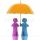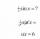# Find the 2

Find the term independent of x in the expansion of (4x3+1/2x)8

Result

x =  7

#### Solution:Leave us a comment of example and its solution (i.e. if it is still somewhat unclear...):

Showing 0 comments:Be the first to comment!#### To solve this example are needed these knowledge from mathematics:

Would you like to compute count of combinations?

## Next similar examples:

1. A studentA student is to answer 8 out of 10 questions on the exam. a) find the number n of ways the student can choose 8 out of 10 questions b) find n if the student must answer the first three questions c) How many if he must answer at least 4 of the first 5 que
2. Parametric equationFind the parametric equation of a line with y-intercept (0,-4) and a slope of -2.
3. Calculation of CNCalculate: ?
4. Fish tankA fish tank at a pet store has 8 zebra fish. In how many different ways can George choose 2 zebra fish to buy?
5. TrinityHow many different triads can be selected from the group 43 students?
6. BlocksThere are 9 interactive basic building blocks of an organization. How many two-blocks combinations are there?
7. CombinationsFrom how many elements we can create 990 combinations 2nd class without repeating?
8. DivisionDivision has 18 members: 10 girls and 6 boys, 2 leaders. How many different patrols can be created, if one patrol is 2 boys, 3 girls and 1 leader?
9. CardsThe player gets 8 cards of 32. What is the probability that it gets a) all 4 aces b) at least 1 ace
10. TeamsHow many ways can divide 16 players into two teams of 8 member?
11. Theorem proveWe want to prove the sentense: If the natural number n is divisible by six, then n is divisible by three. From what assumption we started?
12. BinomialsTo binomial ? add a number to the resulting trinomial be square of binomial.
13. PowersIs true for any number a,b,c equality:? ?
14. Five membersWrite first 5 members geometric sequence and determine whether it is increasing or decreasing: a1 = 3 q = -2
15. Geometric sequence 4It is given geometric sequence a3 = 7 and a12 = 3. Calculate s23 (= sum of the first 23 members of the sequence).
16. GP membersThe geometric sequence has 10 members. The last two members are 2 and -1. Which member is -1/16?
17. GP - 8 itemsDetermine the first eight members of a geometric progression if a9=512, q=2